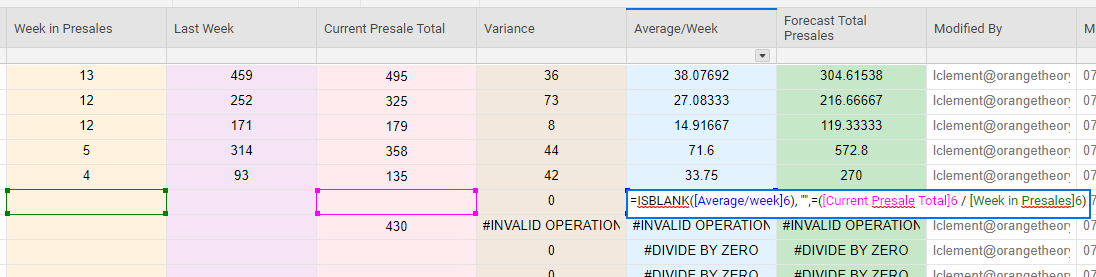# ISBLANK Formula

Hello,l

Im using a simple formula to divide 2 cells, however the forula is showing its dividing by zero in every single cell so im attempting to use an ISBLANK formula so that the formula does not show.

See the screenshot below. My forumula is originally....

=[Current Presale Total]8 / [Week in Presales]8

Then i tried...

=ISBLANK([Average/week]6), "",=([Current Presale Total]6 / [Week in Presales]6) but this isnt working. Can any provide any tips on how to correct this formula.

Thank you!• A couple of things...

It needs to be an IF statement:

=IF(ISBLANK([Average/week]6), "",=([Current Presale Total]6 / [Week in Presales]6)

.

And when nesting a formula within another one, you only need the = at the very beginning (unless you are doing comparisons such as equals, greater than, etc.).

=IF(ISBLANK([Average/week]6), "", [Current Presale Total]6 / [Week in Presales]6)